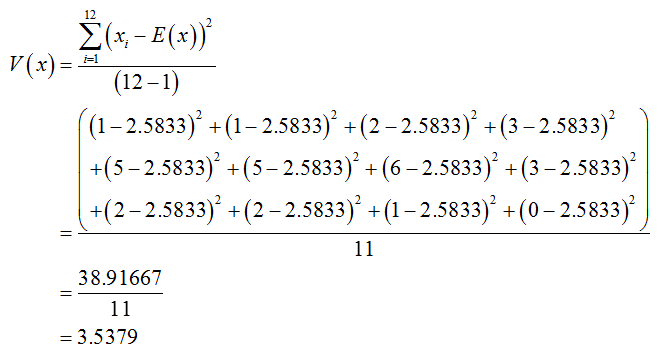# I need a step by step break down on 3 questionsIf the Data set of Mangos are: 1,1,2,3,5,5,6,3,2,2,1,01. E (x^2 -x)2. E(x +3)3 E ( x- x)^2 this question the second x has a bar line above it

Question
3 views

I need a step by step break down on 3 questions
If the Data set of Mangos are: 1,1,2,3,5,5,6,3,2,2,1,0

1. E (x^2 -x)
2. E(x +3)

3 E ( x- x)^2 this question the second x has a bar line above it

check_circle

Step 1

1. Obtain mean of the variable x.

The values of the data set Mangos are:

x: 1, 1, 2, 3, 5, 5, 6, 3, 2, 2, 1 and 0.

Mean:  Mean is an important measure of center when the data is quantitative. Mean of a data set is the sum of the data set divided by the size.

The mean of the variable is obtained as 2.5833 from the calculation given below:

Step 2

Obtain sample variance of the variable x.

The mean of the variable is 2.5833.

The general formula for sample variance is sum of (xi – xbar)^2/(n–1)

The variance of the variable is obtained as 3.5379 from the calculation given below:Step 3

Determine the value of E (x^2 -x):

The general formula for E (x^2 -x) is,

E (x^2 -x) = V(x) + (E(x))^2 – E(x) ; where E(x) is mean and V(x) is variance.

The value of E (x^2 -x) is obtained as 7.628 from the calculation ...

### Want to see the full answer?

See Solution

#### Want to see this answer and more?

Solutions are written by subject experts who are available 24/7. Questions are typically answered within 1 hour.*

See Solution
*Response times may vary by subject and question.
Tagged in

### Other